Civil Created Edited

# What are some precautions to take when generating a mesh for plate elements?

## Question

What are some precautions to take when generating a mesh for plate elements?

1. Density of element mesh

The finer the mesh, the better the results that can be obtained. However, it is not always the case that dividing the mesh into smaller elements is the only solution, as it may significantly increase the analysis time and result interpretation time. Therefore, when generating a mesh for plate elements, it is important to pay attention to the following two items in order to create an appropriate mesh:

▪  For areas where the geometric shape, stiffness (material/thickness), and loading vary, as well as areas where stress concentrations are expected, the mesh should be constructed more densely.
The size difference between adjacent elements should not be excessively large.

There is no exact answer to how finely the mesh should be divided in order to obtain reliable results. As an example, consider the three frame models shown in the figure below. The mesh in the left model is too coarse, while the one on the right is unnecessarily fine.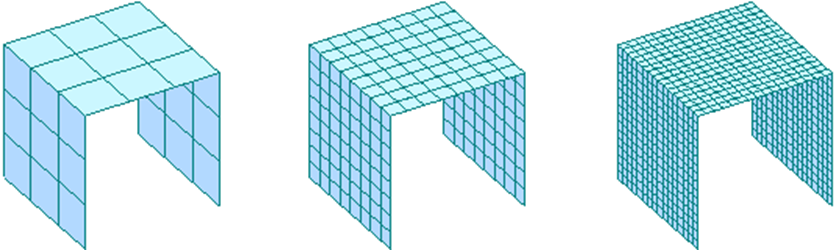If the self-weight is applied to these three models and the displacement is checked, the results are shown in the figure below.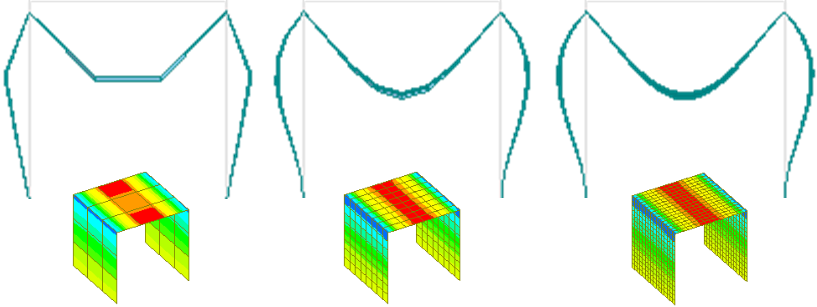It can be observed that distorted stress/member force outputs are produced in models with overly coarse mesh, such as the left model compared to the right model.

2. Shape of element mesh

It is preferable for the mesh size to be small and for the shape of the elements to be close to basic shapes (equilateral triangles, squares). It is better to use square (hexahedral) elements rather than triangular (tetrahedral) elements, and it is better to use triangular elements rather than quadrilateral elements with poor shapes.

1) Circular element mesh

When modeling a cylindrical tank, many users model it with triangles like pizza slices. However, if the elements are divided too finely, one end of the triangle becomes too pointed, resulting in poor quality of the mesh. As mentioned earlier, the quality of the mesh is better when the elements are closer to basic shapes (equilateral triangle, square).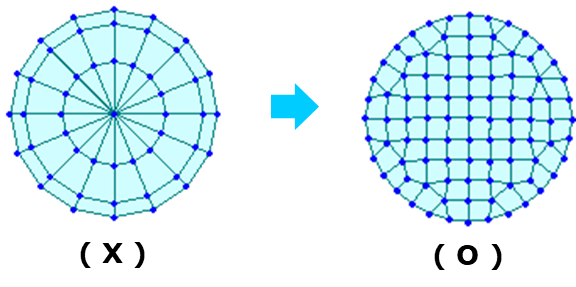Having too many elements connected at a single point is also a factor that lowers the quality of the element mesh.

2) Element mesh with holes

[Structure] tab > [Wizard] group > Base Structure > Plate Wizard function is very limited and cannot be used for various fields. However, it can be used to create a mesh of circular shapes as described earlier and also to create a mesh with holes.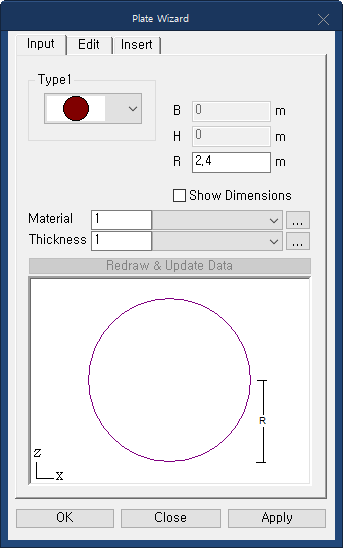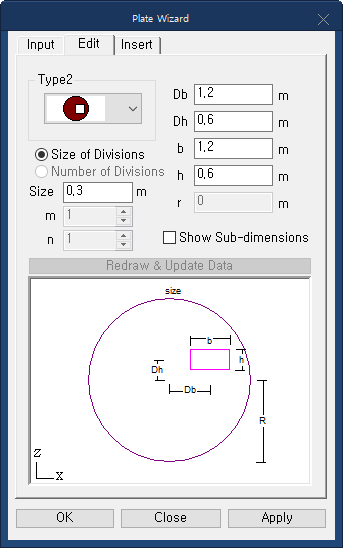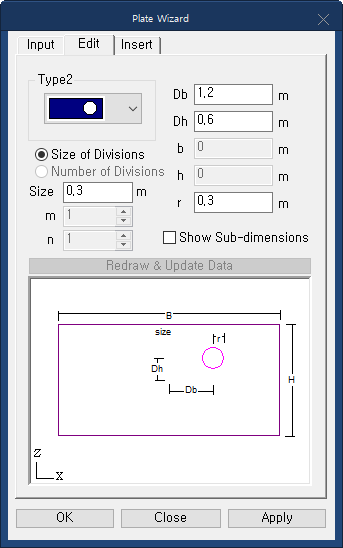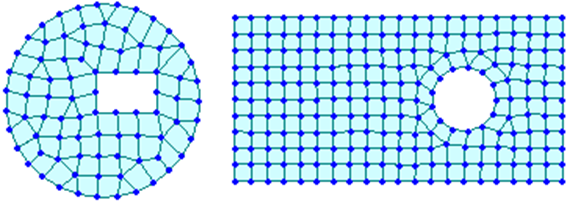3. Dividing element mesh

Areas where loads are applied, sections change, connections with other members are made, and there are supports, should have nodes and should be denser compared to other parts.

In FEA, there are ffunctions such as automatic mesh generation and the ability to adjust the density of the mesh for each part, allowing for more flexible model construction. However, in midas Civil, it is not as easy to model various conditions by adjusting the mesh density.

In the given figure below, in order to ensure that there are nodal points in the necessary parts, all elements present on the plane with nodal points are divided. This type of modeling example can be commonly seen in the surrounding area. Although this type of modeling does not always produce unreliable results, there are some issues, such as: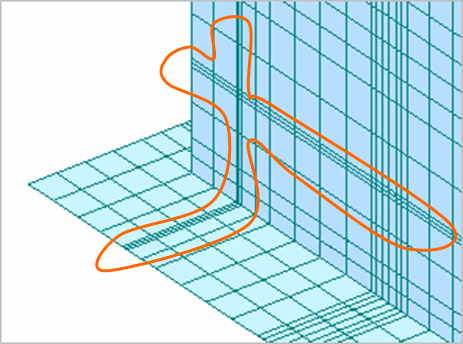• The aspect ratio (the ratio of width to height) is not good.
• The size variation between elements is significant compared to adjacent elements.
• (This is not related to the quality of the element mesh, but) having a large number of elements can result in longer analysis and result verification times.

There are several mesh patterns commonly used in finite element modeling to increase the number of divisions between adjacent elements. Some of them can be utilized through the [Node/Element] tab > [Elenement] group > Divide Element.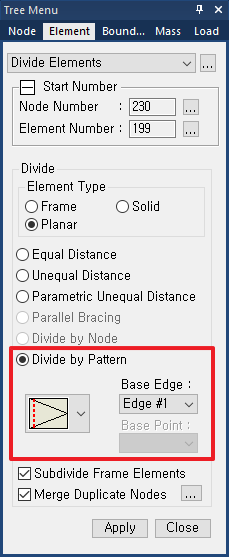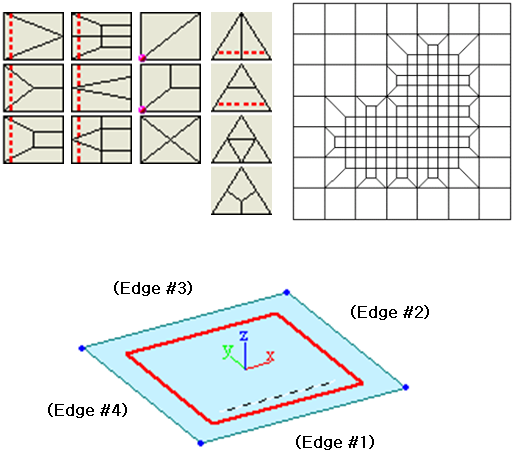.

The 'Edge number' is a unique number determined by the local axis of Plate elements. When selecting an element and an Edge number, it is indicated by a dotted line as shown in the figure above. The number inside the parenthesis is the Edge number when the element coordinate axis is as shown above.

4. Review for element mesh

Let's consider a case where elements overlap as shown in the figure below. Elements 1 and 4 are overlapped at the same location, and element 5 overlaps across elements 2 and 3.1) ShrinkThe Shrink function makes the elements appear to be shrunk or contracted. By using the 'Shrink Elements' function, we can confirm that the 5th element is overlapping, as shown in the image below.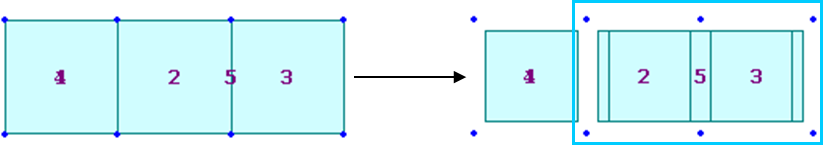2) Delete for duplicate elements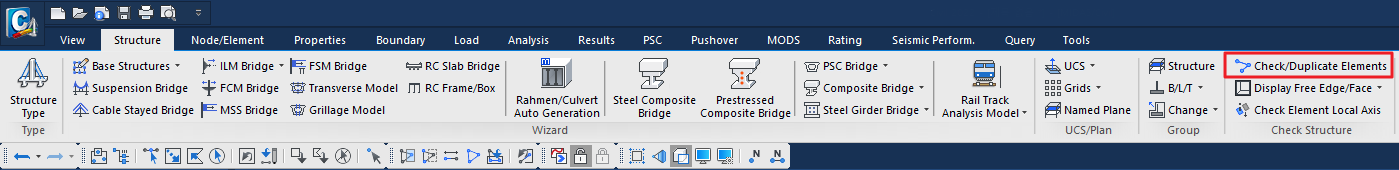You can use the [Structure] tab > [Check Structure] group > Check/Duplicate Elements function to delete duplicate elements that overlap in the same location, keeping only the element with the lowest element number.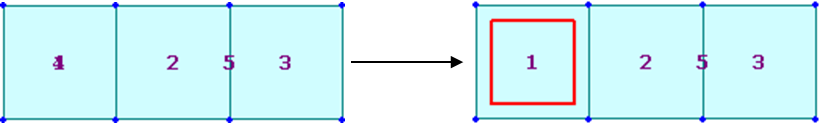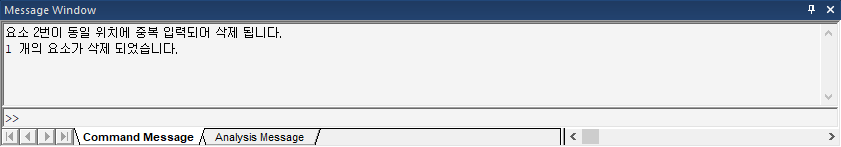3) Check Free Edge

Edges are classified into the following three categories according to their connection state.

• Free Edge : It is not connected with other elements
• Manifold Edge : Two elements are connected
• Non-manifold Edge : More than 3 elements are connected at one edge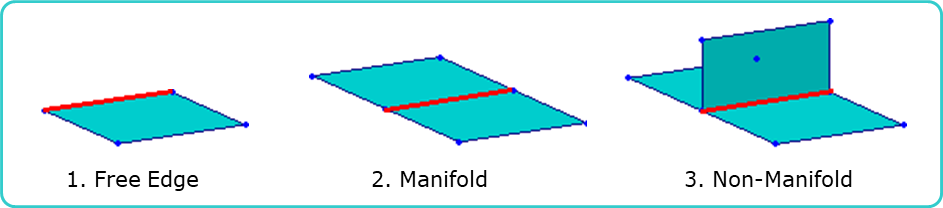By using the [Structure] group > [Check Structure] group > Display Free Edge/Face function, you can check for modeling errors by displaying Free Edges and Faces.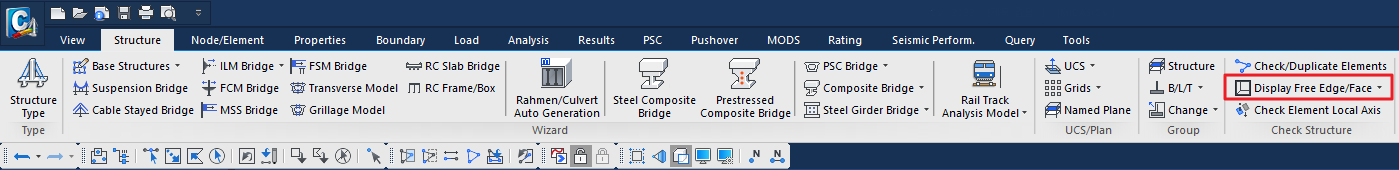The following image is an example of a modeling error commonly made by many users. The edges highlighted in red are Free Edges. In typical cases, only the outer border should be in the Free Edge state, but in this case, there are also Free Edges within the mesh. In such cases, the elements should be divided to prevent the occurrence of Free Edges inside the mesh.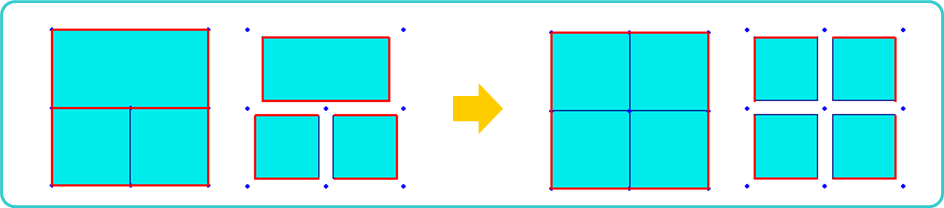4) Merge Nodes

The following figure shows the Free Edges of a storage tank with a hole. While the hole part should be in Free Edge state, it is not appropriate to have Free Edges in the middle of the mesh. If we zoom in on the part where the Free Edge is created, we can see that there are many cases where the nodes are duplicated, as shown in the figure below.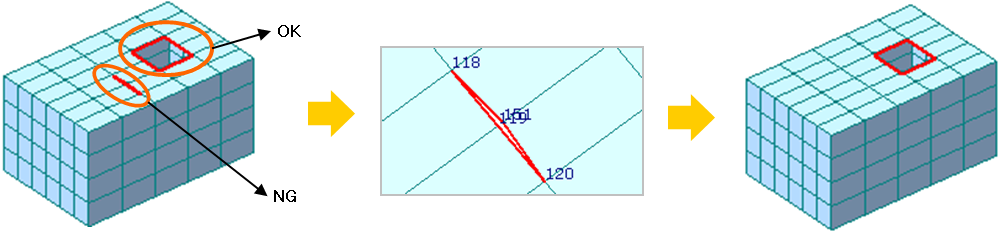In this case, you can use [Node/Element] tab > [Elenement] group > Merge function to combine two nodes into one, thereby eliminating the duplicate nodes and removing the Free Edge that should not exist in the mesh.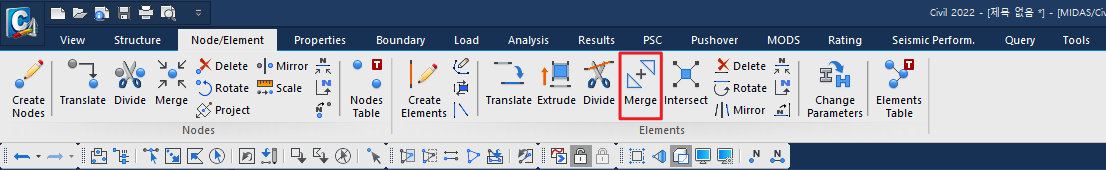0